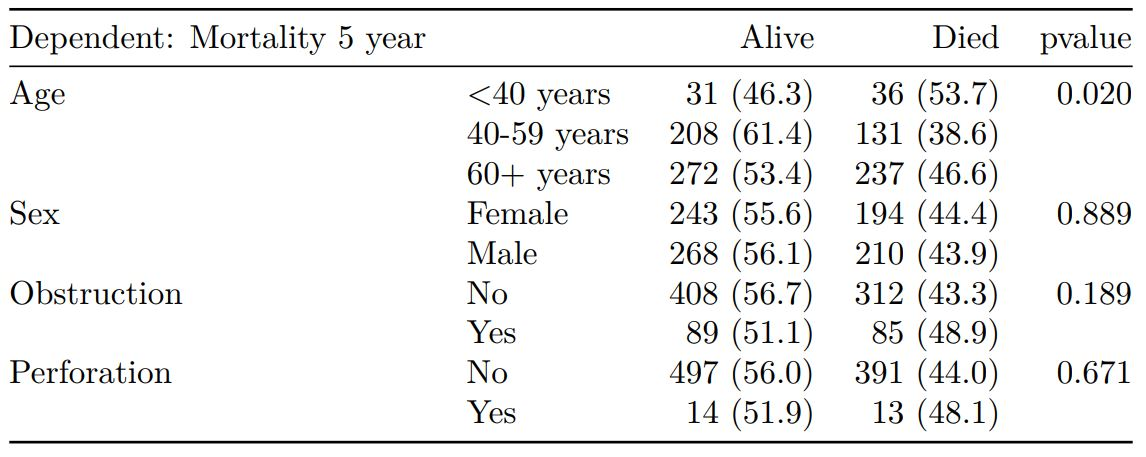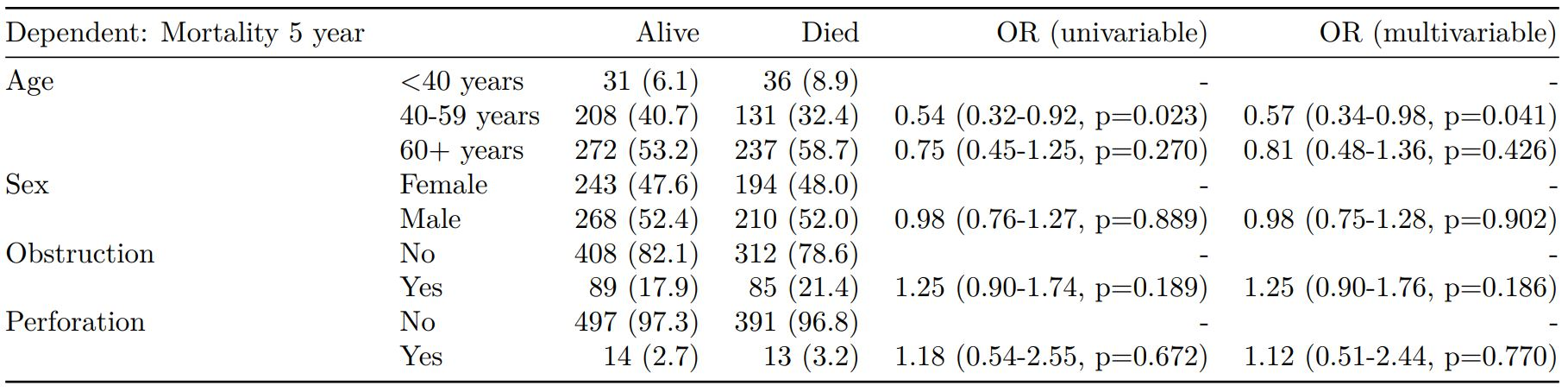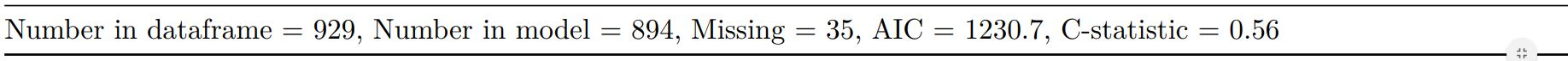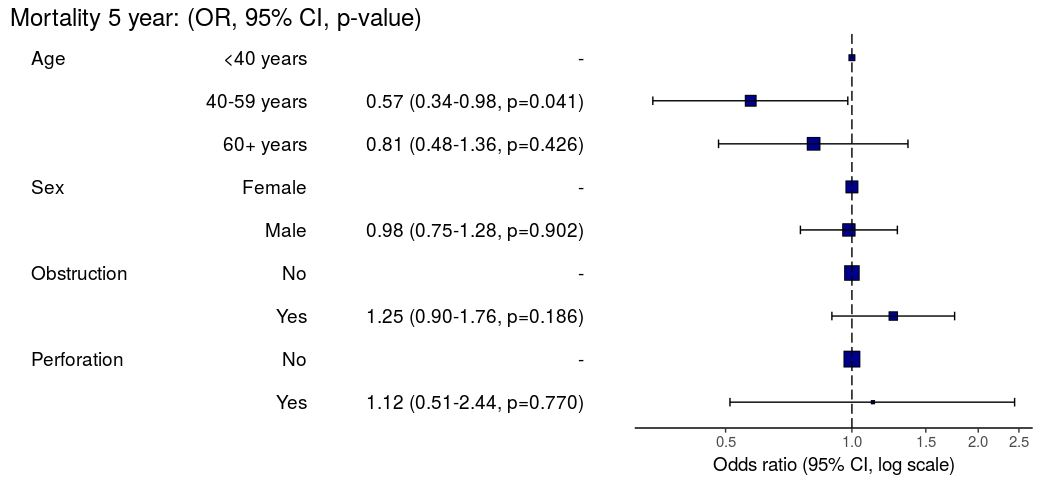The finalfit package provides functions that help you quickly create elegant final results tables and plots when modelling in R. These can easily be exported as Word documents, PDFs, or html files.

Its design follows Hadley Wickham’s tidy tool manifesto.

In addition, it provides functions for identifying and handling missing data, together with a number of functions to bootstrap simulate regression model results.

## Installation

You can install finalfit from CRAN:

install.packages("finalfit")

It is recommended that this package is used together with dplyr which can be installed via:

install.packages("dplyr")

## Documentation

The package documentation is maintained independently at finalfit.org.

## Examples

See getting started and the All tables vignettes for extensive examples.

### Crosstable / table 1

# Crosstable
explanatory = c("age.factor", "sex.factor", "obstruct.factor")
dependent = 'mort_5yr'
colon_s %>%
summary_factorlist(dependent, explanatory,
knitr::kable(t1, align=c("l", "l", "r", "r", "r"))### Regression table

explanatory = c("age.factor", "sex.factor",
"obstruct.factor", "perfor.factor")
dependent = 'mort_5yr'
colon_s %>%
finalfit(dependent, explanatory, metrics=TRUE) -> t2
knitr::kable(t2[], row.names=FALSE, align=c("l", "l", "r", "r", "r", "r"))
knitr::kable(t2[], row.names=FALSE, col.names="")

When exported to PDF:### Regression plots

explanatory = c("age.factor", "sex.factor",
"obstruct.factor", "perfor.factor")
dependent = 'mort_5yr'
colon_s %>%
or_plot(dependent, explanatory)